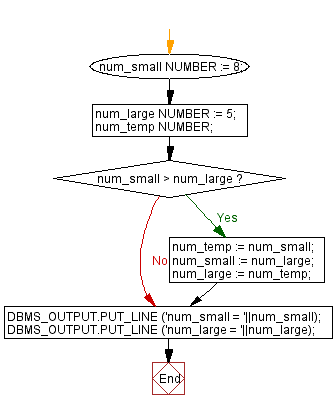﻿ PL/SQL Control Statement: Arrange the number of two variable in such a way that the small number will store in num_small variable and large number will store in num_large variable - w3resource# PL/SQL Control Statement Exercises: Arrange the number of two variable in such a way that the small number will store in num_small variable and large number will store in num_large variable

## PL/SQL Control Statement: Exercise-1 with Solution

Write a PL/SQL program to arrange the number of two variable in such a way that the small number will store in num_small variable and large number will store in num_large variable.

Sample Solution:

PL/SQL Code:

``````DECLARE
num_small NUMBER := 8;
num_large NUMBER := 5;
num_temp NUMBER;
BEGIN

IF num_small > num_large THEN
num_temp := num_small;
num_small := num_large;
num_large := num_temp;
END IF;

DBMS_OUTPUT.PUT_LINE ('num_small = '||num_small);
DBMS_OUTPUT.PUT_LINE ('num_large = '||num_large);
END;
/
``````

Sample Output:

```num_small = 5
num_large = 8
```

Flowchart:Improve this sample solution and post your code through Disqus

What is the difficulty level of this exercise?

﻿